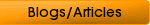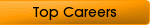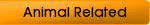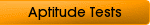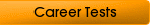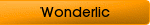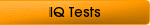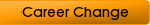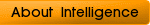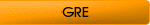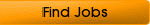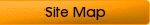:

## Tips Before Taking an Algebra Test

How does one person pass an algebra test and what is it anyway? Many employers now require applicants to pass it because many jobs require basic mathematical skills. A lot of applicants fail on this area and it is therefore important that you prepare yourself before taking it. But how?

Perhaps the most dreaded of all tests is the algebra test. Arithmetic has a certain quality in it that makes people cringe. If you look at it, algebra should be fun. In terms of your career, algebra tests are necessity if you are applying for work that will require mathematical aptitude, even basic ones. Unfortunately, many people fail the test because of lack of understanding.

Algebra is a very important component of many careers and understanding its basic concepts can make a huge impact on your job application. Today, many tests that involve algebra are computerized and in the form of multiple choice. Nevertheless, you need to know the concepts of calculations to get the right answer.

### Algebra Defined

Just what exactly is algebra? Simply defined, it is a specific branch of mathematics. The difference is that it uses statements to make it easier to describe the differences or similarities between things. This means that variables, represented by letters, are used to describe these relationships. Perhaps you remember the things you learned in high school or college when you were given algebraic expressions such as n and x or y. Anything that has these figures are examples of algebra.

### Algebraic Terms, Equations, and Expressions

Algebraic phrases are also called terms, equations, and expressions. An algebra test will contain many terms and expressions so you need to be able to define what these things are. Simply put, a term is a number. This could also refer to the product of a number and a variable or a letter. An example of an algebraic term is -3ax. In this term, there is something called the numerical coefficient, which is described as the part of the term that has a number. In this example, the numerical coefficient is -3. The variables, on the other hand, are the terms ax.

In an algebra test, you will also come across a collection of numbers, which is called an expression. These are combinations of numbers and variables that contain algebraic terms, signs of basic mathematical operations such as subtraction, multiplication, division, or addition. A key tip to remember is that an algebraic expression does not contain an equal sign (=). A great example is -3ax + 11yz.

The last kind of algebraic statement you need to know is an equation. An equation exists if two algebraic expressions are equal to something. An example is 4 + 9 = 13. The first expression is 4. The second expression is +9. Since these two combined is equal to nine, it makes an equation. Remember that an equation will always have an equal sign (=). Essentially, an algebraic equation tells you that if your variables are correct, you will always arrive to the same conclusion.

### How to Pass the Test

Passing the test is the ultimate challenge. Many times, you find yourself unprepared for these tests so we have a few tips here for you. The first thing you need to have to pass an algebra test is confidence. Since all of the things you will take in the test are things you have already learned in school, you just have to review and be confident that you can make it.

One key factor is to pass the test is to change your perspective towards numbers. Many people are put off when they begin seeing variables or letters in equations. If you would only start looking at these letters as opportunities to make variations, then you are more likely to get it in your system faster.

If you are taking a tutorial, use flashcards. These cards help you learn quicker because they encourage visual learning. It helps you remember the terms and numbers and convert the memory into your own writing later on.

If you are reviewing for the exam, we recommend that you use college study guides. Many study guides and reviewers are available in reputable bookstores, complete with instructions. A review book before your algebra test will help you prepare in scoring higher.

If you are having trouble understanding the basic concepts of algebra and solving equations or expressions, perhaps you can start working on your skills with someone who is already or currently learning it. Sure enough, you know someone in your neighborhood who is currently taking a class in algebra who can help you.

Home
Careers in Math
Math Aptitude Test
Numerical Test
Quantitative Aptitude Test
Numerical Aptitude Tests